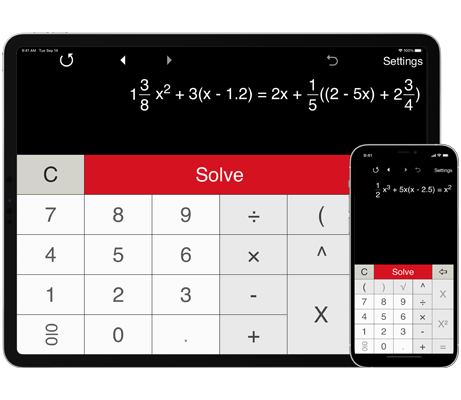# Linear equation solver

Linear Equation Solver with steps to solve linear equations with one variable.
Shows step-by-step solution.
Supports fractions and parentheses.

## Linear equation solver with steps

Solves linear equations with integers.

Solves linear equations with decimals.

Solves linear equations with fractions.

Solves linear equations with brackets and parentheses.

Provides special formats for solving first degree equations in the form
ax + b = c
and
ax + b = cx + d.